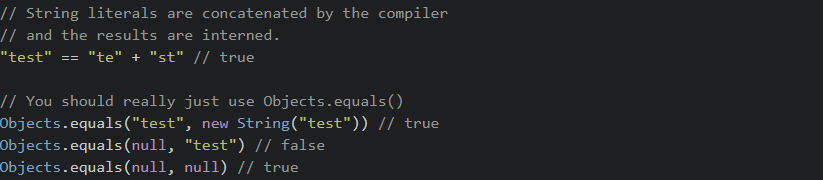# Java - How do I compare strings?Using the `==` operator to compare strings is common but can have some pitfalls. Below is a short example of the difference between .equals and == operators and when it's appropriate to use them.

`==` tests for reference equality (if they are the same object).

`.equals()` tests for value equality (if they are logically "equal").

Objects.equals() checks for `null` before calling `.equals()` so you don't have to.

String.contentEquals() compares the content of the `String` with the content of any `CharSequence`.

Therefore, if you want to test if two strings have the same value you will probably want to use `Objects.equals()`.

``````// These two have the same value
new String("test").equals("test") // true

// But they are not the same object
new String("test") == "test" // false

// Neither are these
new String("test") == new String("test") // false

// These are because literals are interned by
// the compiler and thus refer to the same object
"test" == "test" // true

// String literals are concatenated by the compiler
// and the results are interned.
"test" == "te" + "st" // true

// You should really just use Objects.equals()
Objects.equals("test", new String("test")) // true
Objects.equals(null, "test") // false
Objects.equals(null, null) // true``````

You should always use `Objects.equal``s()`. Unless you are In a situation where you're dealing with interned strings, then use the `== operator.`

Moreover, a string literal always refers to the same instance of class `String`. This is because string literals - or, more generally, strings that are the values of constant expressions (§15.28) - are "interned" so as to share unique instances, using the method `String.intern`.

There is also plenty of good examples on SO, check this query,

shop